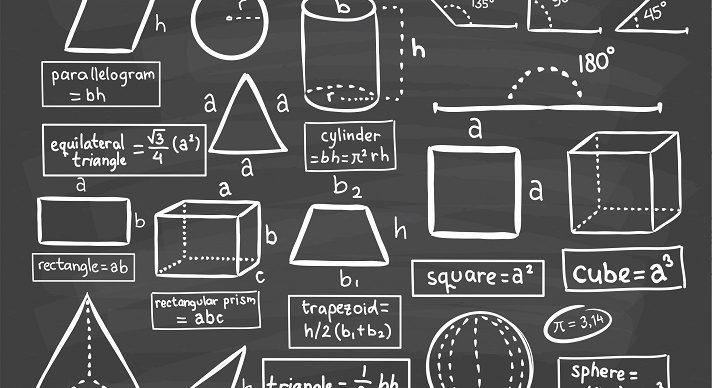### Course Overview

In the Acellus Grade 7 Math course, after a thorough review of basic operations, Ms. Frogge demonstrates how to solve real-world problems by applying basic concepts of Algebra and Geometry and by using proportional relationships. Students are also shown how to use statistical data to make inferences and how to use and evaluate probability models.

### Course Objectives & Student Learning Outcomes

Upon completion of Acellus Grade 7 Math, students will be able to: compare integers; choose opposites; use absolute value; add integers on integer Hill, using a Chip Model and on a number line; subtract, multiply, and divide integers; use order of operations including with integers; interpret, compare, estimate, round, add, subtract, multiply, and divide decimals by a whole number, by a decimal; and converting percents to decimals; find greatest common factor and least common multiple; identify and reduce fractions; convert mixed to improper fractions, improper to mixed fractions, fractions to terminating decimals, fractions to repeating decimals; order rational numbers; find common denominator; add and subtract simple fractions; add and subtract mixed numbers; add and subtract mixed numbers with regrouping; multiply simple fractions and mixed numbers; divide simple fractions and mixed numbers; add and subtract rational numbers; and multiply and divide negative fractions.

Students will also be able to read and write expressions and equations; evaluate expressions; simplify expressions with integers; add and subtract rational coefficients; simplify, expand, factor, identify, and translate expressions; write equations; solve one-step and two-step equations; solve equations with rational numbers and with variables on both sides; write and graph inequalities; solve inequalities; solving inequality in word problems; identify and find adjacent complementary, supplementary, reflex, and vertical angles and angels that share a vertex; draw triangles and angels; find area of parallelograms, triangles and trapezoids; define circle vocabulary; find circumference, radius, diameter, and area of circles; find area of irregular figures; identify 3D figures, and nets; relate cross sections; calculate surface area of prisms, pyramids, cylinders and composite figures; calculate volume of prisms, cylinders, pyramids and composite figures.

Finally, students will be able to calculate ratios, proportions, unit rate, proportions with fractions, proportions and percents; implement scale factor; calculate length given a scale; calculate areas in scale drawings; calculate proportionality in tables, graphs, verbal descriptions and equations; interpret graphs; calculate inverse proportionality in tables, in graphs, and in verbal descriptions; calculate mode, range; create Box and Whisker Plots; identify random sampling and compare data sets; utilize probability models of categorized data and with frequencies; calculate theoretical and experimental probability; identify, compare, and contrast independent and dependent events.

### Scope and Sequence

Unit 1 – Integers This unit discusses comparing, adding, subtracting, and multiplying integers, including the concepts of opposites, absolute value, and order of operations. Unit 2 – Decimals This unit covers Interpreting, comparing, estimating with, rounding, adding, subtracting, and multiplying decimals, as well as dividing decimals by whole numbers and by decimals, and converting percents to decimals. Unit 3 – Fraction Basics This unit discusses greatest common factors, least common multiples, what fractions mean, how to reduce them, how to convert mixed fractions to improper fractions and vice versa, how to convert fractions to terminating and to repeating decimals, and ordering rational numbers. Unit 4 – Fraction Operations This unit covers finding a common denominator, then delves into adding, subtracting, multiplying, and dividing simple fractions, mixed numbers, rational numbers, and negative fractions. Unit 5 – Fraction Expressions This unit presents algebraic vocabulary regarding expressions and equations, evaluating expressions, simplifying and expanding expressions with integers and rational numbers, adding and subtracting rational coefficients, factoring algebraic expressions, recognizing equivalent expressions, and translating expressions. Unit 6 – Equations and Inequalities This unit covers writing equations, solving one- and two-step equations and equations with rational numbers and with variables on both sides. It also covers writing, graphing, and solving inequalities, as well as inequality word problems. Unit 7 – Angles This unit discusses adjacent, complementary, supplementary, reflex, and vertical angles, as well as angles that share a vertex, triangles and angles, and drawing triangles. Unit 8 – 2D Figures This unit discusses area of parallelograms, triangles, and trapezoids, area and circumference and of circles, and area of irregular figures. Unit 9 – 3D Figures This unit covers identifying 3D figures and nets, cross sections, and surface area and volume of prisms, pyramids, cylinders, and composite figures. Unit 10 – Rates and Ratios This unit discusses ratios, basics of proportions and proportions with fractions and percents, unit rate, scale factor, using a scale to calculate length, areas in scale drawings, and scaled drawings with different scales. Unit 11 – Proportionality This unit covers direct proportionality in tables, graphs, verbal descriptions, and equations, and inverse proportionality in tables, graphs, and verbal descriptions. Unit 12 – Statistics and Probability This unit discusses mean, mode, median and range, box and whisker plots, random sampling, comparing data sets, probability models with categorical data and with frequencies, theoretical and experimental probability, and independent and dependent events.This course was developed by the International Academy of Science. Learn More